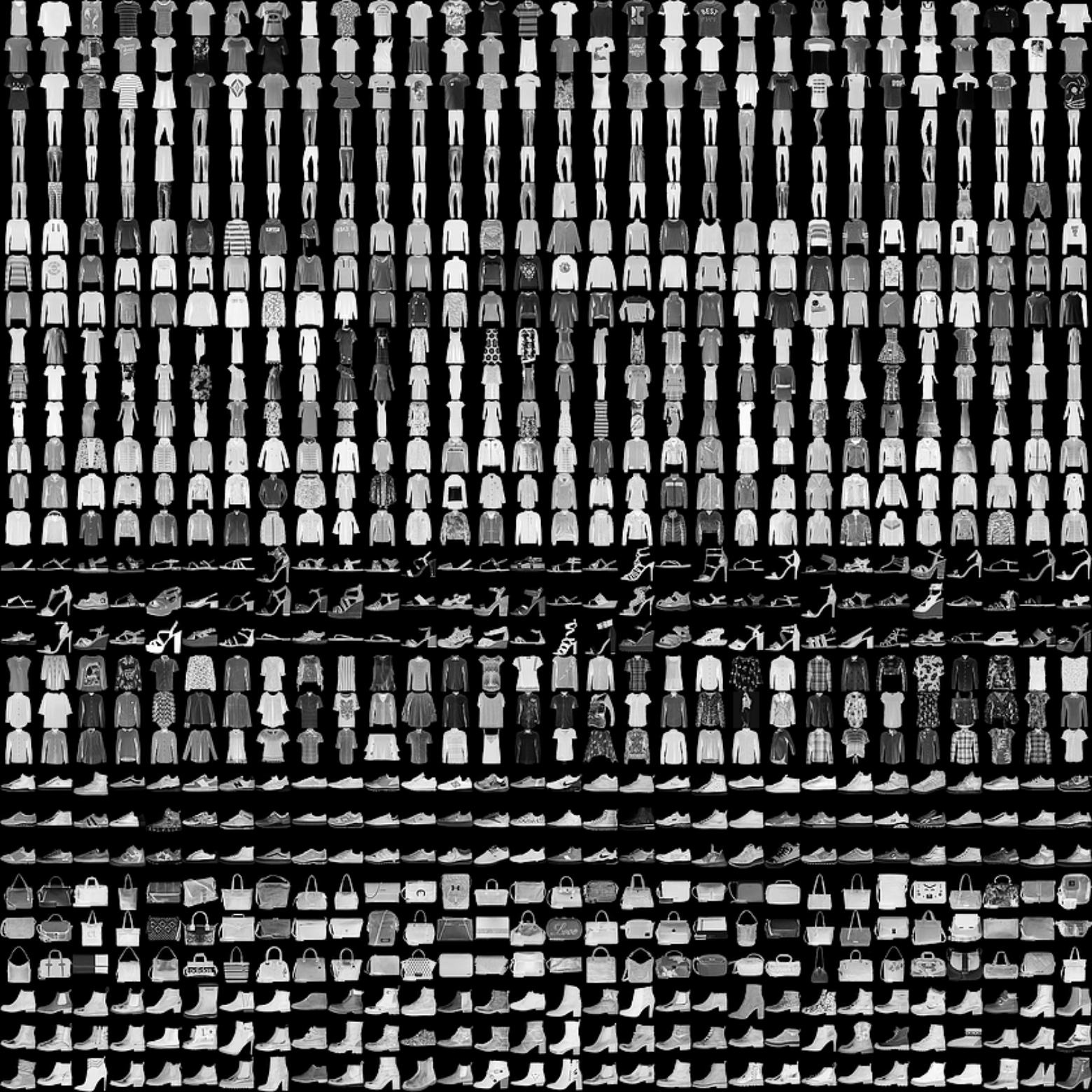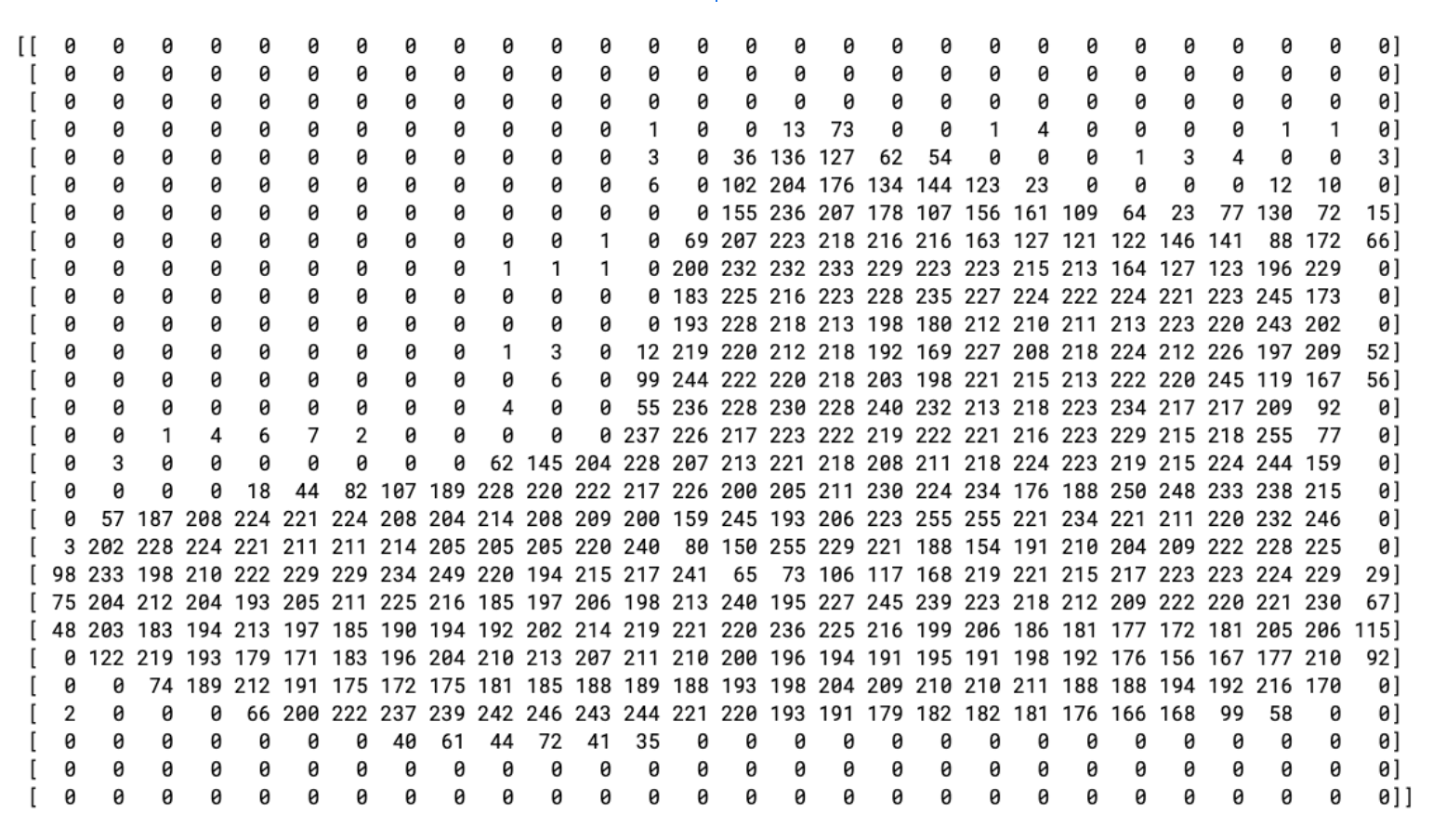使用 TensorFlow 构建计算机视觉模型

前提条件

• 扎实的 Python 知识
• 基本编程技能

学习内容

• 训练神经网络识别衣物
• 完成一系列练习，这些练习会引导您对不同的神经网络层进行实验

• 一个可识别衣物的神经网络

2. 开始编码

import tensorflow as tf
print(tf.__version__)标签 说明 0 T 恤衫/上衣 1 裤子 2 套衫 3 裙子 4 外套 5 凉鞋 6 衬衫 7 运动鞋 8 包 9 踝靴

Fashion MNIST 数据可在 tf.keras.datasets API 中获取。加载方式如下所示：

mnist = tf.keras.datasets.fashion_mnist

(training_images, training_labels), (test_images, test_labels) = mnist.load_data()

import matplotlib.pyplot as plt
plt.imshow(training_images)
print(training_labels)
print(training_images)training_images  = training_images / 255.0
test_images = test_images / 255.0

3. 设计模型

model = tf.keras.models.Sequential([tf.keras.layers.Flatten(),
tf.keras.layers.Dense(128, activation=tf.nn.relu),
tf.keras.layers.Dense(10, activation=tf.nn.softmax)])
• Sequential 定义了神经网络中的层序列。
• Flatten 会接受一个正方形并将其转换为一维矢量。
• Dense 会添加一层神经元。
• Activation 函数会告知各层神经元要执行的操作。选项有很多，但目前只采用以下选项：
• Relu 实际上意味着，如果 X 大于 0，则返回 X，否则返回 0。它只会将 0 或更大的值传递到网络中的下一层。
• Softmax 会接受一组值，并能有效地选择最大的值。例如，如果最后一层的输出是 [0.1, 0.1, 0.05, 0.1, 9.5, 0.1, 0.05, 0.05, 0.05]，那么您无需通过排序来获取最大值，它会返回 [0,0,0,0,1,0,0,0,0]。

4. 编译和训练模型

loss = 'sparse_categorical_crossentropy',
metrics=['accuracy'])

model.fit(training_images, training_labels, epochs=5)

model.fit 执行时，您会看到损失和准确率：

Epoch 1/5
60000/60000 [=======] - 6s 101us/sample - loss: 0.4964 - acc: 0.8247
Epoch 2/5
60000/60000 [=======] - 5s 86us/sample - loss: 0.3720 - acc: 0.8656
Epoch 3/5
60000/60000 [=======] - 5s 85us/sample - loss: 0.3335 - acc: 0.8780
Epoch 4/5
60000/60000 [=======] - 6s 103us/sample - loss: 0.3134 - acc: 0.8844
Epoch 5/5
60000/60000 [=======] - 6s 94us/sample - loss: 0.2931 - acc: 0.8926

5. 测试模型

model.evaluate(test_images, test_labels)

10000/10000 [=====] - 1s 56us/sample - loss: 0.3365 - acc: 0.8789
[0.33648381242752073, 0.8789]

练习 1

classifications = model.predict(test_images)
print(classifications)

练习 6

import tensorflow as tf
print(tf.__version__)
mnist = tf.keras.datasets.fashion_mnist
(training_images, training_labels), (test_images, test_labels) = mnist.load_data()
#training_images=training_images/255.0
#test_images=test_images/255.0
model = tf.keras.models.Sequential([
tf.keras.layers.Flatten(),
tf.keras.layers.Dense(512, activation=tf.nn.relu),
tf.keras.layers.Dense(10, activation=tf.nn.softmax)
])
model.fit(training_images, training_labels, epochs=5)
model.evaluate(test_images, test_labels)
classifications = model.predict(test_images)
print(classifications)
print(test_labels)

7. 探索回调

import tensorflow as tf

class myCallback(tf.keras.callbacks.Callback):
def on_epoch_end(self, epoch, logs={}):
if(logs.get('accuracy')>0.95):
print("\nReached 95% accuracy so cancelling training!")
self.model.stop_training = True

callbacks = myCallback()
mnist = tf.keras.datasets.fashion_mnist
(training_images, training_labels), (test_images, test_labels) = mnist.load_data()
training_images=training_images/255.0
test_images=test_images/255.0
model = tf.keras.models.Sequential([
tf.keras.layers.Flatten(),
tf.keras.layers.Dense(512, activation=tf.nn.relu),
tf.keras.layers.Dense(10, activation=tf.nn.softmax)
])